Function Repository Resource:

# FactorialMod

Fast factorial modulo a given number

Contributed by: Aster Ctor
 ResourceFunction["FactorialMod"][n,p] computes a fast version of Mod[Factorial[n],p].

## Details and Options

ResourceFunction["FactorialMod"] accelerates its computiation using the Number Theoretic Transform (NTT).

## Examples

### Basic Examples (2)

Compute a factorial modulo 53:

 In:=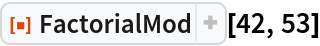Out=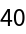Check the result:

 In:=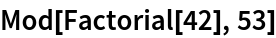Out=### Scope (4)

Work with a nonprime modulus:

 In:=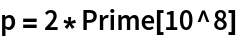Out=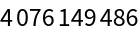The simple algorithm is slow:

 In:=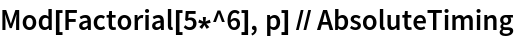Out=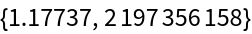A native speed up is two times faster:

 In:=Out=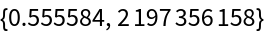NTT speed up is four times faster:

 In:=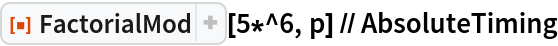Out=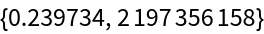## Publisher

Aster Ctor (MoeNet)

## Version History

• 1.0.0 – 23 October 2019